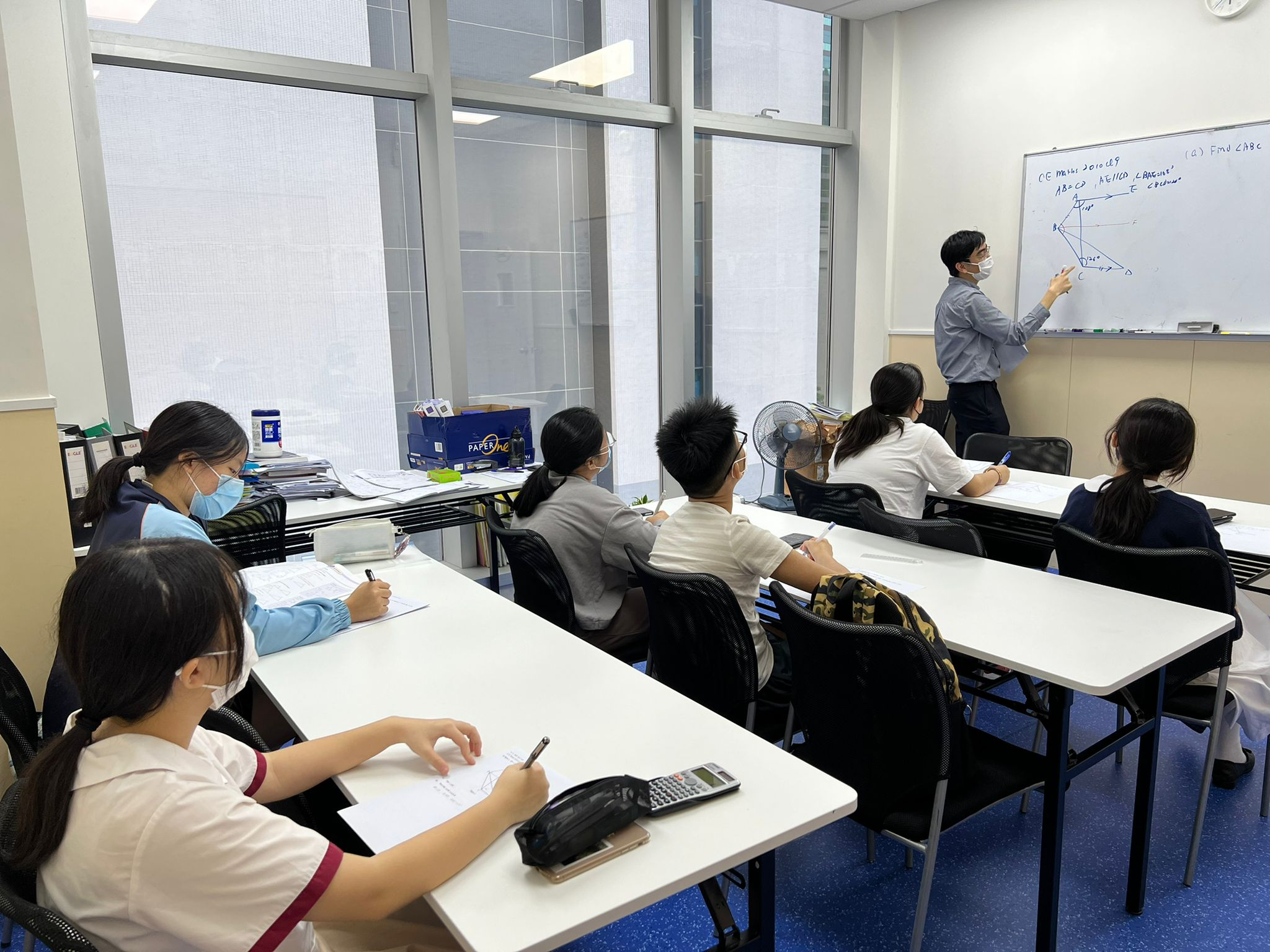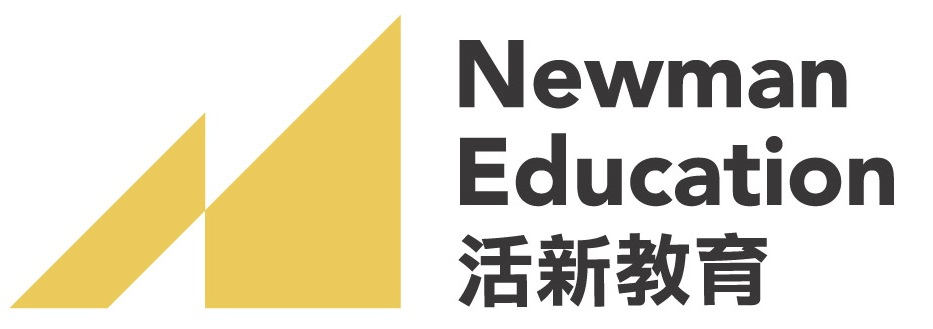## 幼兒課程### 中一學習關鍵：打好代數基本功

Learning Key: To lay a solid foundation of Algebra

### 中二學習關鍵：全面提升代數概念

Learning Key: To help cultivate a sense and concept of Algebra

### 中三學習關鍵：DSE試題初體驗

Learning Key: To first experience the DSE questions

### 數學常規課程時間表及報名表

#### Subject List (3 Subjects)

• 1. Number and Algebra
1. Basic computation 基礎計算 2. Directed numbers 有向數 3. Approximate values and numerical estimation 近似值與數值估算 4. Rational and irrational numbers 有理數與無理數 5. Using percentages 百分法 6. Rates, ratios and proportions 率、比及比例 7. Algebraic expressions 代數式 8. Linear equations in one unknown 一元一次方程 9. Linear equations in two unknowns 二元一次方程 10. Laws of integral indices 整數指數律 11. Polynomials 多項式 12. Identities 恆等式 13. Formulae 公式 14. Linear inequalities in one unknown 一元一次不等式
• 2. Measures, Shape and Space 度量、圖形與空間
1. Errors in measurement 量度的誤差 2. Arc lengths and areas of sectors 弧長和扇形面積 3. 3-D figures 立體圖形 4. Mensuration 求積法 5. Angles and parallel lines 角和平行線 6. Polygons 多邊形 7. Congruent triangles 全等三角形 8. Similar triangles 相似三角形 9. Quadrilaterals 四邊形 10. Centres of triangles 三角形的心 11. Pythagoras’ theorem 畢氏定理 12. Rectangular coordinate system 直角坐標系 13. Trigonometry 三角學
• 3. Data Handling 數據處理
1. Organisation of data 數據的組織 2. Presentation of data 數據的表達 3. Measures of central tendency 集中趨勢的度量 4. Probability 概率

#### Course Teacher (1 Teacher)### 陳SIR